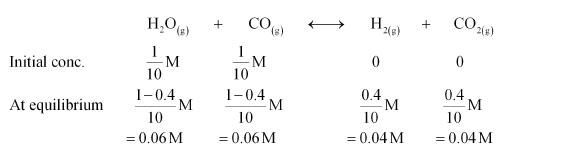# One mole of H2O and one mole of CO are taken in 10 L vessel and heated to`
Question:

One mole of $\mathrm{H}_{2} \mathrm{O}$ and one mole of $\mathrm{CO}$ are taken in $10 \mathrm{~L}$ vessel and heated to

725 K. At equilibrium 40% of water (by mass) reacts with CO according to the equation,

$\mathrm{H}_{2} \mathrm{O}(\mathrm{g})+\mathrm{CO}(\mathrm{g}) \longleftrightarrow \mathrm{H}_{2}(\mathrm{~g})+\mathrm{CO}_{2}(\mathrm{~g})$

Calculate the equilibrium constant for the reaction.

Solution:

The given reaction is:Therefore, the equilibrium constant for the reaction,

$K_{\mathrm{C}}=\frac{\left[\mathrm{H}_{2}\right]\left[\mathrm{CO}_{2}\right]}{\left[\mathrm{H}_{2} \mathrm{O}\right][\mathrm{CO}]}$

$=\frac{0.04 \times 0.04}{0.06 \times 0.06}$

$=0.444$ (approximately)